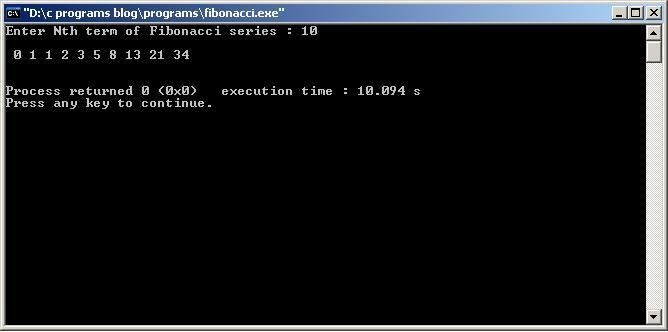# C program to print Fibonacci series upto N th term

/* C program to generate and print Fibonacci series */
```#include<stdio.h>
main()
{
int n,i,a=0,b=1,c=0;
printf("Enter Nth term of Fibonacci series : ");
scanf("%d",&n);
printf("\n %d %d ",a,b); //for printing first two numbers
for(i=2;i<n;i++)  // for printing from 3rd number in series
{
c=a+b;
a=b;
b=c;
printf("%d ",c);
}
printf("\n\n");
return(0);
}```
Output: ( using GNU GCC Compiler with Code Blocks IDE )